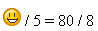Subject: Honors Algebra 2 Name: Jill Who are you: Parent How do we algebraically figure out how many M&Ms are in a container? Hi Jill. There are several ways, but it depends on your measurement approach. If you are allowed length measurements, you can find the dimensions of the container and calculate the volume. Then you can find the dimensions of one candy and find its volume. If you divide, you will get the number of candies, except that the answer will be too high because the shape of the candies ensures that there are air pockets inside as well. If you make the simplification that the candies are box-shaped and find their volume from that, then you'll get an answer too low, but you'll at least have a range in which the true answer lies. Algebraically, you'd define lengths and heights and depths and volumes using variable names like a, b, c, and v. You can set up equations with these values (and of course the formulas for the volume calculations) to produce your answer. Here's an example: say the container is a box-shape with length a, height b, depth c and volume v. Then v = abc. Let's say we simplify and say that a candy is box shaped with length and width both x and thickness y, and volume z. Then z = xxy. So the number of candies would be v/z. If you expand that, it is (abc)/(xxy). When I read Sue's solution I thought that there would probably still be air spaces between the candies and (abc)/(xxy) would give too many candies. You might pour water into the container full of M&Ms then pour it out and measure the volume w of water used as a measure of the air space. Then the number of candies would be (abc-w)/(xxy). Unfortunately you would probably end up with soggy M&Ms. Penny If you are allowed mass (weight) measurements, then you can weigh an empty container, weigh a single candy and weigh the container full of candies. For example, if the empty container weighs e grams, the full container weighs f grams and one candy weighs c grams, then the number of candies is (f - e) / c. Remember that in algebra you can assign variable names in any fashion that helps you remember what the variable represents. Once on an assignment we had a question where we had to calculate the number of jokes a stand-up comedian could tell in 5 minutes if she could tell 80 jokes in 8 minutes. I decided to use happy faces for the variable name (instead of j, which most other students used).Always remember to have fun when you're playing with math! Stephen La Rocque.